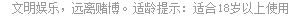# 升级中副牌牌力控制点

副牌牌力的计算极为复杂，现在介绍比较轻易明白和实用的一种。

A=2.5点， 对AA=6点。（A A 1）

K=1.5点， 对KK=4点。（K K 1）

QJ105=0.5点， 对QJ105=2点。

(拖拉机忽略不计，拖拉机另外计算，最高计算为8点）

AKJJ9854=7.5点。KJJ9854=4.5点。

AKJJ985=7点。 JJ9854=2.5点。

AKJJ98=6点。 J9854=1.5点。

AKJJ9=6.5点。 9854=1.5点。

AKJJ=7点。 854=2点。

AKJ=6点。 54=2.5点。

AK=6点。 4=2.5点。

A=5点。 绝=6点。

• [07-05]
• [12-06]
• [10-27]
• [12-05]
• [06-21]
• [11-24]
• [02-23]
• [01-26]
• [01-26]
• [01-26]

## 客服中心

4007202233(9:00-21:00) 在线客服

## 常见问题